﻿ Numerical Implementation of MCC and GCC Models | Nonlinear Models | Online Help | GEO5
0Tree
Settings
Program:
Language:

## Numerical Implementation of MCC and GCC Models

An important step, ensuring a reliable application of MCC and GCC models, is the determination of the initial preconsolodation pressure pcin and the corresponding unit modulus Kin. These two parameters, however, are not directly specified by the user. Instead, they are derived by the program based on the assumed distribution of initial geostatic stress. Recall three basic options to derive the intial geostatic stress:

1. Using Ko procedure

The use of Ko procedure yields the following value of the initial mean stress:where: Ko - coefficient of earth pressure at rest γ - unit weight of soil h - current depth below terrain

Assuming normal consolidation, the value of pcin is determined such that the stress derived using the Ko procedure fullfils the yield condition:where: Mcs - slope of critical state line J - equivalent deviatoric stress σm - mean stress

Values of J and σm are defined by the following expressions: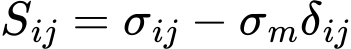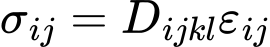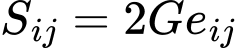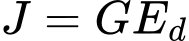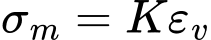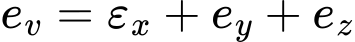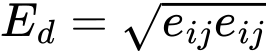where: Ed - equivalent deviatoric stress eij - deviatoric strain tensor εij - overall strain tensor εv - volumetric strain σij - stress tensor sij - deviatoric stress tensor δij - Kronecker's delta Dijkl - elastic stiffness tensor G - elastic shear modulus K - elastic unit modulus E - Young's modulus ν - Poisson's ratio

In case of triaxial compression or extension it is possible to determine the slope of critical state line Mcs from the following expressions:In case of overconsolidated soils the initial value of pcin is modified as: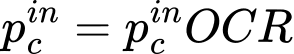The initial value of the unit modulus follows from:where the current void ratio e is written as:For small stresses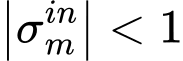we get:## 2. Standard (elastic) analysis

Recall that the program allows for replacing the material model between stages of construction. Providing the Ko procedure cannot be used it is possible to carry out the analysis assuming elastic response of the clayey soil. The resulting stresses are used to derive the initial values of pcin and Kin employing the previously defined expressions.  In the next stages of construction the original elastic material model is replaced by the required MCC or GCC models.

## 3. Standard (plastic) analysis

This option allows the soil to be consolidated under the assumption of nonlinear behavior when generating the geostatic stress. This results in the evolution of plastic strains already in the first stage of construction. As in the Ko procedure we consider a normally consolidated soil which, during the course of deformation, moves down the normal consolidation line with the initial values of pcin and Kin given by: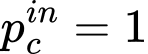Before the next analysis step the resulting plastic strains are set equal to zero. In some cases such an analysis may fail to converge.